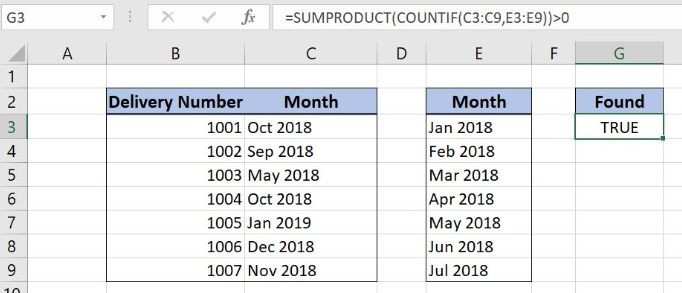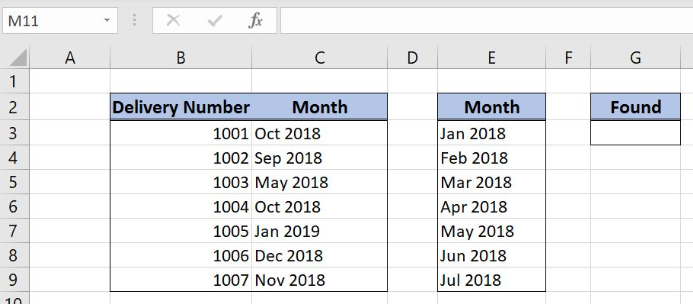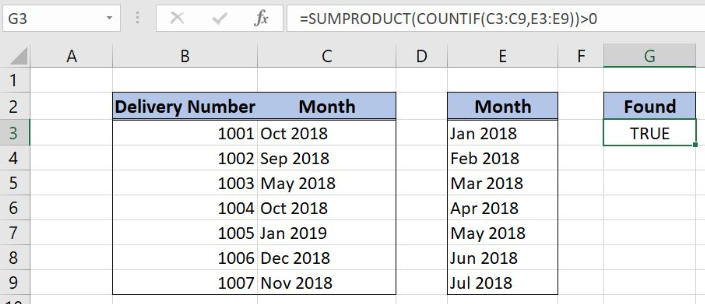Get instant live expert help with Excel or Google Sheets“My Excelchat expert helped me in less than 20 minutes, saving me what would have been 5 hours of work!”

#### Post your problem and you'll get expert help in seconds

Your message must be at least 40 characters
Our professional experts are available now. Your privacy is guaranteed.

# Range contains one of many values

Excel allows a user to check if a range contains one of many values from the other range, using the SUMPRODUCT and COUNTIF functions. This step by step tutorial will assist all levels of Excel users in comparing two ranges.Figure 1. The result of the formula

## Syntax of the COUNTIF Formula

The generic formula for the COUNTIF function is:

`=COUNTIF(criteria_range, criteria)`

The parameters of the COUNTIF function are:

• criteria_range – a range where we want to apply our criteria
• criteria – a criteria which we want to count.

## Syntax of the SUMPRODUCT Formula

`=SUMPRODUCT(--(array))`

The parameters of the SUMPRODUCT function for counting with criteria are:

• array – an array of values which we want to sum

## Setting up Our Data for the FormulaFigure 2. Data that we will use in the example

Let’s look at the structure of the data we will use. We want to check if at least of the values from column C appears in column E. The formula returns True or False in the cell G3.

## Checking if the Range Contains One of Many Values

The formula looks like:

`=SUMPRODUCT(COUNTIF(C3:C9, E3:E9)) > 0`

The criteria_range of the COUNTIF function is the C3:C9 range, while the criteria is E3:E9. The result of the function is the array parameter of the SUMPRODUCT function. Finally, the function sums all these values and check if the sum is greater than 0.

To apply the formula, we need to follow these steps:

• Select cell G3 and click on it
• Insert the formula: `=SUMPRODUCT(COUNTIF(C3:C9,E3:E9))>0`
• Press enterFigure 3. Using the formula to check ranges matching

As we can see in Figure 3, the result of the formula is TRUE. The value in the C5 (May 2018) appear in the range E3:E9.

Most of the time, the problem you will need to solve will be more complex than a simple application of a formula or function. If you want to save hours of research and frustration, try our live Excelchat service! Our Excel Experts are available 24/7 to answer any Excel question you may have. We guarantee a connection within 30 seconds and a customized solution within 20 minutes.

### Did this post not answer your question? Get a solution from connecting with the expert.Another blog reader asked this question today on Excelchat:
Related blogs## Subscribe to Excelchat.coAnother blog reader asked this question today on Excelchat: